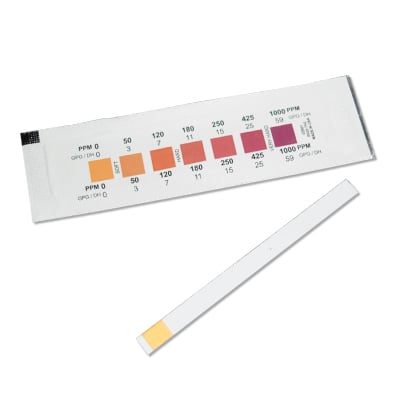# Question 49362

Feb 28, 2017

The hardness of the water is 1350 ppm.

#### Explanation:

In water testing, paper strips often measure hardness in parts per million (ppm), where 1 ppm is defined as 1 mg of ${\text{CaCO}}_{3}$ per litre of water.Let's first calculate the concentration of ${\text{Ca(OH)}}_{2}$ in parts per million.

["Ca(OH)"_2] = (1000 color(red)(cancel(color(black)("g"))))/(1 color(red)(cancel(color(black)("m"^3)))) ×"1000 mg"/(1 color(red)(cancel(color(black)("g")))) × (1 color(red)(cancel(color(black)("m"^3))))/"1000 L" = "1000 mg/L" = "1000 ppm"#

The molar mass of ${\text{Ca(OH)}}_{2}$ is 74.09 g/mol, and the molar mass of ${\text{CaCO}}_{3}$
is 100.09 g/mol.

Thus, we need 100.09 g of ${\text{CaCO}}_{3}$ to get the same number of calcium ions as there in 74.09 g of ${\text{Ca(OH)}}_{2}$.

$\text{Hardness" = "1000 ppm" × 100.09/74.09 = "1350 ppm}$

This is VERY hard water. It would be off the scale on the test paper and give a deep purple colour.